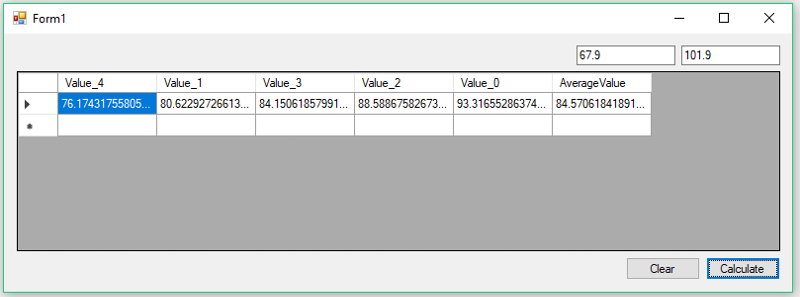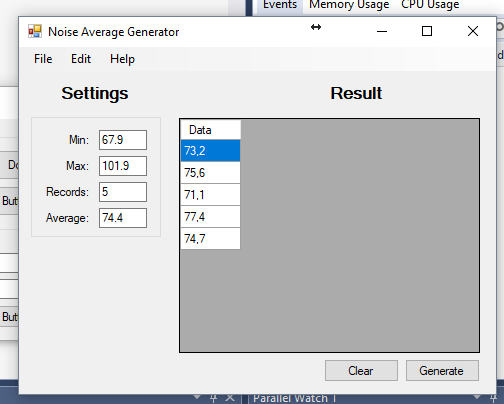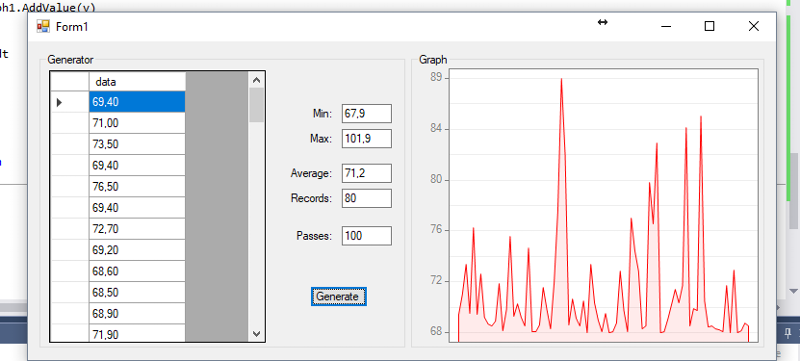# [VB.NET] Generate Random Numbers by Given Range and Given Average

Hello Experts!

I'm trying to do a little function for my app, but i really don't know to do it.

Example:

I want to do a function where i give it a range (Min / Max), Average and How many records like:

Min: 67.9
Max: 101.9
Records: 5
Average: 74.4

So it should generate into my DataGridView1 (Or DataTable) 5 records of numbers with a final average of 74.4.

The Result:
``````73.2
75.6
71.1
77.4
74.7

Average: 74.4
``````

How can i do this?
Network ManagerAsked:
###### Who is Participating?
I wear a lot of hats...

"The solutions and answers provided on Experts Exchange have been extremely helpful to me over the last few years. I wear a lot of hats - Developer, Database Administrator, Help Desk, etc., so I know a lot of things but not a lot about one thing. Experts Exchange gives me answers from people who do know a lot about one thing, in a easy to use platform." -Todd S.

Analyst Assistant Commented:
So you want to generate n numbers within a range and the average of those should match a given number?

Are there any constraints other than the max/min?
Network ManagerAuthor Commented:
Exactly!

That's what it should do, probably later i would try something to smooth the numbers so it wouldn't be far from each other because it will be a graph, but for now i'm just focusing in generate "n" numbers and the average of the range should match the given number. (Which is important).
DeveloperCommented:
Datagrids and Datatables are better for column based datasets.  That being said, here is a quick application to get you started:

Form1.cs -
``````using System;
using System.Collections.Generic;
using System.Data;
using System.Linq;
using System.Windows.Forms;

namespace EE_Q29071125
{
public partial class Form1 : Form
{
public Form1()
{
InitializeComponent();
}

private void OnLoad(object sender, EventArgs e)
{
dataGridView1.Enabled = false;
button1.Enabled = false;
textBox2.Enabled = false;
}

private void OnClick(object sender, EventArgs e)
{
if (sender is Button)
{
var btn = sender as Button;
if (btn.Equals(button1))
{
double first = Convert.ToDouble(textBox1.Text);
double second = Convert.ToDouble(textBox2.Text);
var set = (from i in Enumerable.Range(0, 5)
let value = (first < second) ? RandomProvider.Next(first, second) : RandomProvider.Next(second, first)
orderby value
select new KeyValuePair<string, double>(\$"Value_{i}", value)).ToDictionary(k => k.Key, v => v.Value).AddAverageColumn();

var table = new DataTable("Range");
table.Columns.AddRange(set.Select(c => new DataColumn { ColumnName = c.Key, DataType = typeof(double) }).ToArray());
table.Rows.Add(set.Select(c => c.Value).Cast<object>().ToArray());
dataGridView1.DataSource = table;
dataGridView1.Enabled = true;
}
else if (btn.Equals(button2))
{
dataGridView1.DataSource = null;
dataGridView1.Enabled = false;
button1.Enabled = false;
textBox2.Enabled = false;
textBox1.Clear();
textBox2.Clear();
}
}
}

private void OnValidating(object sender, System.ComponentModel.CancelEventArgs e)
{
if (sender is TextBox)
{
var tb = sender as TextBox;
double value = -1d;
if (double.TryParse(tb.Text, out value))
{
if (tb.Equals(textBox1))
{
textBox2.Enabled = true;
}
else if (tb.Equals(textBox2))
{
button1.Enabled = true;
}
}
else
{
if (!string.IsNullOrWhiteSpace(tb.Text))
{
e.Cancel = true;
tb.Clear();
tb.Focus();
MessageBox.Show("You must insert a valid value");
}
}
}
}
}

static class Extensions
{
public static Dictionary<string, double> AddAverageColumn(this Dictionary<string, double> source)
{
source.Add("AverageValue", source.Values.Average());
return source;
}
}

/// <summary>
/// RandomProvider.  Provides random numbers of all data types
/// in specified ranges.  It also contains a couple of methods
/// from Normally (Gaussian) distributed random numbers and
/// Exponentially distributed random numbers.
/// </summary>
/// <remarks>Original implementation - https://www.cambiaresearch.com/articles/13/csharp-randomprovider-class
/// </remarks>
public class RandomProvider
{
private static Random m_RNG1;
private static double m_StoredUniformDeviate;
private static bool m_StoredUniformDeviateIsGood = false;

#region -- Construction/Initialization --

static RandomProvider()
{
Reset();
}
public static void Reset()
{
m_RNG1 = new Random(Environment.TickCount);
}

#endregion

#region -- Uniform Deviates --

/// <summary>
/// Returns double in the range [0, 1)
/// </summary>
public static double Next()
{
return m_RNG1.NextDouble();
}

/// <summary>
/// Returns true or false randomly.
/// </summary>
public static bool NextBoolean()
{
if (m_RNG1.Next(0, 2) == 0)
return false;
else
return true;
}

/// <summary>
/// Returns double in the range [0, 1)
/// </summary>
public static double NextDouble()
{
double rn = m_RNG1.NextDouble();
return rn;
}

/// <summary>
/// Returns Int16 in the range [min, max)
/// </summary>
public static Int16 Next(Int16 min, Int16 max)
{
if (max <= min)
{
string message = "Max must be greater than min.";
throw new ArgumentException(message);
}
double rn = (max * 1.0 - min * 1.0) * m_RNG1.NextDouble() + min * 1.0;
return Convert.ToInt16(rn);
}

/// <summary>
/// Returns Int32 in the range [min, max)
/// </summary>
public static int Next(int min, int max)
{
return m_RNG1.Next(min, max);
}

/// <summary>
/// Returns Int64 in the range [min, max)
/// </summary>
public static Int64 Next(Int64 min, Int64 max)
{
if (max <= min)
{
string message = "Max must be greater than min.";
throw new ArgumentException(message);
}

double rn = (max * 1.0 - min * 1.0) * m_RNG1.NextDouble() + min * 1.0;
return Convert.ToInt64(rn);
}

/// <summary>
/// Returns float (Single) in the range [min, max)
/// </summary>
public static Single Next(Single min, Single max)
{
if (max <= min)
{
string message = "Max must be greater than min.";
throw new ArgumentException(message);
}

double rn = (max * 1.0 - min * 1.0) * m_RNG1.NextDouble() + min * 1.0;
return Convert.ToSingle(rn);
}

/// <summary>
/// Returns double in the range [min, max)
/// </summary>
public static double Next(double min, double max)
{
if (max <= min)
{
string message = "Max must be greater than min.";
throw new ArgumentException(message);
}

double rn = (max - min) * m_RNG1.NextDouble() + min;
return rn;
}

/// <summary>
/// Returns DateTime in the range [min, max)
/// </summary>
public static DateTime Next(DateTime min, DateTime max)
{
if (max <= min)
{
string message = "Max must be greater than min.";
throw new ArgumentException(message);
}
long minTicks = min.Ticks;
long maxTicks = max.Ticks;
double rn = (Convert.ToDouble(maxTicks)
- Convert.ToDouble(minTicks)) * m_RNG1.NextDouble()
+ Convert.ToDouble(minTicks);
return new DateTime(Convert.ToInt64(rn));
}

/// <summary>
/// Returns TimeSpan in the range [min, max)
/// </summary>
public static TimeSpan Next(TimeSpan min, TimeSpan max)
{
if (max <= min)
{
string message = "Max must be greater than min.";
throw new ArgumentException(message);
}

long minTicks = min.Ticks;
long maxTicks = max.Ticks;
double rn = (Convert.ToDouble(maxTicks)
- Convert.ToDouble(minTicks)) * m_RNG1.NextDouble()
+ Convert.ToDouble(minTicks);
return new TimeSpan(Convert.ToInt64(rn));
}

/// <summary>
/// Returns double in the range [min, max)
/// </summary>
public static double NextUniform()
{
return Next();
}

/// <summary>
/// Returns a uniformly random integer representing one of the values
/// in the enum.
/// </summary>
public static int NextEnum(Type enumType)
{
int[] values = (int[])Enum.GetValues(enumType);
int randomIndex = Next(0, values.Length);
return values[randomIndex];
}

#endregion

#region -- Exponential Deviates --

/// <summary>
/// Returns an exponentially distributed, positive, random deviate
/// of unit mean.
/// </summary>
public static double NextExponential()
{
double dum = 0.0;
while (dum == 0.0)
dum = NextUniform();
return -1.0 * System.Math.Log(dum, System.Math.E);
}

#endregion

#region -- Normal Deviates --

/// <summary>
/// Returns a normally distributed deviate with zero mean and unit
/// variance.
/// </summary>
public static double NextNormal()
{
// based on algorithm from Numerical Recipes
if (m_StoredUniformDeviateIsGood)
{
m_StoredUniformDeviateIsGood = false;
return m_StoredUniformDeviate;
}
else
{
double rsq = 0.0;
double v1 = 0.0, v2 = 0.0, fac = 0.0;
while (rsq >= 1.0 || rsq == 0.0)
{
v1 = 2.0 * Next() - 1.0;
v2 = 2.0 * Next() - 1.0;
rsq = v1 * v1 + v2 * v2;
}
fac = System.Math.Sqrt(-2.0
* System.Math.Log(rsq, System.Math.E) / rsq);
m_StoredUniformDeviate = v1 * fac;
m_StoredUniformDeviateIsGood = true;
return v2 * fac;
}
}
#endregion
}
}
``````
Form1.Designer.cs -
``````namespace EE_Q29071125
{
partial class Form1
{
/// <summary>
/// Required designer variable.
/// </summary>
private System.ComponentModel.IContainer components = null;

/// <summary>
/// Clean up any resources being used.
/// </summary>
/// <param name="disposing">true if managed resources should be disposed; otherwise, false.</param>
protected override void Dispose(bool disposing)
{
if (disposing && (components != null))
{
components.Dispose();
}
base.Dispose(disposing);
}

#region Windows Form Designer generated code

/// <summary>
/// Required method for Designer support - do not modify
/// the contents of this method with the code editor.
/// </summary>
private void InitializeComponent()
{
this.textBox1 = new System.Windows.Forms.TextBox();
this.textBox2 = new System.Windows.Forms.TextBox();
this.button1 = new System.Windows.Forms.Button();
this.dataGridView1 = new System.Windows.Forms.DataGridView();
this.button2 = new System.Windows.Forms.Button();
((System.ComponentModel.ISupportInitialize)(this.dataGridView1)).BeginInit();
this.SuspendLayout();
//
// textBox1
//
this.textBox1.Location = new System.Drawing.Point(579, 12);
this.textBox1.Name = "textBox1";
this.textBox1.Size = new System.Drawing.Size(100, 20);
this.textBox1.TabIndex = 0;
this.textBox1.Validating += new System.ComponentModel.CancelEventHandler(this.OnValidating);
//
// textBox2
//
this.textBox2.Location = new System.Drawing.Point(685, 12);
this.textBox2.Name = "textBox2";
this.textBox2.Size = new System.Drawing.Size(100, 20);
this.textBox2.TabIndex = 1;
this.textBox2.Validating += new System.ComponentModel.CancelEventHandler(this.OnValidating);
//
// button1
//
this.button1.Location = new System.Drawing.Point(710, 226);
this.button1.Name = "button1";
this.button1.Size = new System.Drawing.Size(75, 23);
this.button1.TabIndex = 3;
this.button1.Text = "Calculate";
this.button1.UseVisualStyleBackColor = true;
this.button1.Click += new System.EventHandler(this.OnClick);
//
// dataGridView1
//
this.dataGridView1.ColumnHeadersHeightSizeMode = System.Windows.Forms.DataGridViewColumnHeadersHeightSizeMode.AutoSize;
this.dataGridView1.Location = new System.Drawing.Point(13, 38);
this.dataGridView1.Name = "dataGridView1";
this.dataGridView1.Size = new System.Drawing.Size(772, 182);
this.dataGridView1.TabIndex = 2;
//
// button2
//
this.button2.Location = new System.Drawing.Point(629, 226);
this.button2.Name = "button2";
this.button2.Size = new System.Drawing.Size(75, 23);
this.button2.TabIndex = 4;
this.button2.Text = "Clear";
this.button2.UseVisualStyleBackColor = true;
this.button2.Click += new System.EventHandler(this.OnClick);
//
// Form1
//
this.AutoScaleDimensions = new System.Drawing.SizeF(6F, 13F);
this.AutoScaleMode = System.Windows.Forms.AutoScaleMode.Font;
this.ClientSize = new System.Drawing.Size(797, 261);
this.Controls.Add(this.button2);
this.Controls.Add(this.button1);
this.Controls.Add(this.dataGridView1);
this.Controls.Add(this.textBox2);
this.Controls.Add(this.textBox1);
this.Name = "Form1";
this.Text = "Form1";
this.Load += new System.EventHandler(this.OnLoad);
((System.ComponentModel.ISupportInitialize)(this.dataGridView1)).EndInit();
this.ResumeLayout(false);
this.PerformLayout();

}

#endregion

private System.Windows.Forms.TextBox textBox1;
private System.Windows.Forms.TextBox textBox2;
private System.Windows.Forms.Button button1;
private System.Windows.Forms.DataGridView dataGridView1;
private System.Windows.Forms.Button button2;
}
}
``````
Which produces the following output --saige-
High School Computer Science, Computer Applications, Digital Design, and Mathematics TeacherCommented:
Thinking out loud algorithm...

1) Fill a List with X numbers at exactly your desired Average.
2) For each Value, pick a direction (up/down) to move, and pick a distance that keeps it within the min/max values.
3) Pick a different random value and move it the opposite direction by that distance, as long as it stays within the min/max values.
4) Repeat numbers 2 and 3 for all values several times.
Network ManagerAuthor Commented:
@It_saige
Well i'm writing it in vb.net, what i'm trying to do is exactly what Mike mentioned, but still, really thank you for the help!!

@Mike
This is a little visual example of what i'm trying to do.High School Computer Science, Computer Applications, Digital Design, and Mathematics TeacherCommented:
I'm at work right now so I can't code. If no one has satisfactorily answered by the time I settle down this evening, ill see if I can whip something up.
Analyst Assistant Commented:
Giulia

Whenever the Generate button is pressed do you want to, well, generate a new set of n numbers that fit the criteria?
Network ManagerAuthor Commented:
Yes!,  whenever i press the generate button, it does use the settings on the left.
High School Computer Science, Computer Applications, Digital Design, and Mathematics TeacherCommented:
Here's something to play with, definitely could use some improvement, but I'm tired:
``````    Private Sub Button1_Click(sender As Object, e As EventArgs) Handles Button1.Click
DataGridView1.DataSource = GenData(67.9, 101.9, 74.4, 5, 100)
End Sub

Private Function GenData(ByVal min As Double, ByVal max As Double, ByVal average As Double, ByVal numRecords As Integer, ByVal numPasses As Integer) As DataTable
Static rnd As New Random

Dim j As Integer
Dim delta As Double
Dim directionDown As Boolean
Dim values As New List(Of Double)

Dim dt As New DataTable
dt.Columns.Add(New DataColumn("data", GetType(Double)))
For i As Integer = 1 To numRecords
values.Add(average)
Next

For pass As Integer = 1 To numPasses
For i As Integer = 0 To values.Count - 1
directionDown = rnd.NextDouble() < 0.5
If directionDown Then
delta = (values(i) - min) * rnd.NextDouble
Else
delta = (max - values(i)) * rnd.NextDouble
End If
Do
j = rnd.Next(values.Count)
Loop While (j = i)
If directionDown Then
If values(j) + delta <= max Then
values(i) = values(i) - delta
values(j) = values(j) + delta
End If
Else
If values(j) - delta >= min Then
values(i) = values(i) + delta
values(j) = values(j) - delta
End If
End If
Next
Next
For Each v As Double In values
dt.Rows.Add(v)
Next
Return dt
End Function
``````

Experts Exchange Solution brought to you by

Your issues matter to us.

Facing a tech roadblock? Get the help and guidance you need from experienced professionals who care. Ask your question anytime, anywhere, with no hassle.

Network ManagerAuthor Commented:
@Mike Tomlinson

Congratulations!!!

That's exactly what i need, i have been testing it, i'm using this to make a Personal protective equipment (PPE) attenuation Simulator to get a random average attenuation so i can export the generated data and import into a software. Is there a way to add another condition so it would insert random peak values (a little high values) ? If not that's pretty okay!

Here is the result of the Function into my code.High School Computer Science, Computer Applications, Digital Design, and Mathematics TeacherCommented:
"Is there a way to add another condition so it would insert random peak values (a little high values) ?"

I'm sure it's possible...should there be a corresponding low value?...or should the difference be spread out among several other values?  How many peaks should there be?  Do you want one, two, or three?...or maybe calculate a low percentage of peak values.  Does a peak value come within X% of the max?...or does it exceed the max?  So many questions and ways to do this...
Network ManagerAuthor Commented:
Ok, let's see:

1) Should there be a corresponding low value? or should the difference be spread out among several other values?

The difference should be spread out among several other values.

2) How many peaks should there be?  Do you want one, two, or three?...or maybe calculate a low percentage of peak values.
I guess if it calculates a low percentage of peak values could be awesome!

3) Does a peak value come within X% of the max?...or does it exceed the max?
No, it shouldn't exceed the maximum value given. but there could be a little percentage it could reach the max or somewhat near.

I guess with these conditions it should generate a consistent "Average Noise" data simulation.

I'm really happy with the results so far, Thank you!
Network ManagerAuthor Commented:
Even though the topic was not finished, Thank you a lot @Mike for helping me with the app and @it_saige for giving it a start.
###### It's more than this solution.Get answers and train to solve all your tech problems - anytime, anywhere.Try it for free Edge Out The Competitionfor your dream job with proven skills and certifications.Get started today Stand Outas the employee with proven skills.Start learning today for free Move Your Career Forwardwith certification training in the latest technologies.Start your trial today
Visual Basic.NET

From novice to tech pro — start learning today.